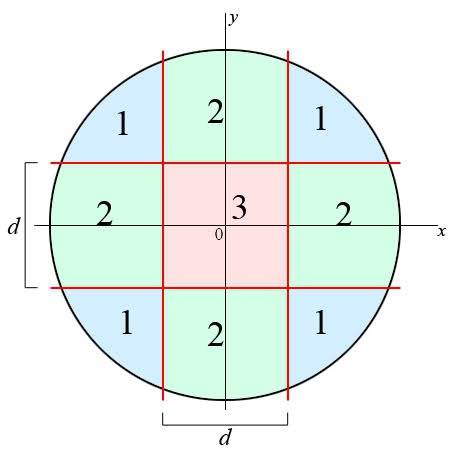# Divide circle into 9 areas

• Nick89
In summary, the conversation discusses trying to find the optimal distance d for dividing a circle with a radius of 32 units into 9 equal areas. The first approach is to divide the total area by 9 and equal it to d^2, but this does not yield equal areas. The next approach involves calculating the areas using integrals and trying to minimize the deviation between the areas. However, this also does not result in equal areas. The question is where the mistake was made and if there is a solution where all areas are equal.

#### Nick89

Hi,

I was asked this question on another forum and was interested in it... It's somewhat related to what I have been doing lately so I gave it a (few) tries, but I never really worked it out...

Consider a circle with a radius of 32 units. We want to divide the area of the circle into 9 areas that have, if possible, exactly the same area. See the following image:The red lines are the 'dividing lines', spaced by a distance $d$ (in the x as well as the y direction).

The areas 1 (blue) and 2 (green) and the area 3 (red) are marked with the colors. Note that there are four areas 1 and four areas 2, they should be equal in area.

The question is how to find the distance $d$ that will yield the optimal result (if possible, that all areas are equal).

The first thing I thought about (but which doesn't seem to be working, see later) is simply to do the following:

We know the area of the complete circle: $$A_{tot} = \pi 32^2$$
Therefore, if the 9 areas are to be divided in equal areas, the area of one the subareas will be: $$A_{sub} = \frac{ \pi 32^2}{9}$$
We also know the area $A_3$ since it's just a square: $$A_3 = d^2$$
Therefore: $$d = \sqrt{ \frac{ \pi 32^2}{9}}$$.

I tried to graph it and it seemed alright to the eye, but I wanted to be sure, so I went on...

The following way I could think of was to calculate the subareas seperately using integrals and then looking for a $d$ that would minimize their deviation.

I came up with the following area's; $A_1$ is calculated from the top-right area1 and $A_2$ is calculated from the rightmost area2.

$$A_1 = \int_\frac{d}{2}^b \left( \sqrt{ 1024-x^2} - \frac{d}{2} \right) \, dx$$
$$A_2 = 2 \times \left( \int_b^{32} \sqrt{1024-x^2} \, dx \right) + d \sqrt{1024-\frac{d^2}{4}}$$
$$A_3 = d^2$$
where the limit b is the intersection of the circle with y = d/2:
$$b = \sqrt{1024-\frac{d^2}{4}}$$

When I now plugged in the value for $d$ I found above I don't get the same result, I get a different result for each area...

So, I thought, maybe my simple solution above wasn't right.
But now I have found three areas each as a function of d. I should be able to minimize the deviation between the areas for one value of d, right? I can't see any way how to do that though... Maybe taking the absolute value of the deviation (A_1 - A_2 for example) and using solving it's derivative for 0? Even then I only minimized A_1 - A_2 and had nothing to do with A_3...

Where have I gone wrong:
1) Assuming there is a solution where all areas are equal;
2) Assuming this solution was simply to divide the total area by 9 and equaling this to d^2;
3) Calculating the areas using integrals?

I can't see any other mistakes I may have made, so I assume it must be one of the three...

Could anyone help me out here?

I would calculate the areas (with integrals as needed) as a function of d, then try to minimize
$$4(A_1-A)^2+4(A_2-A)^2+(A_3-A)^2$$ with $$A=1024\pi/9$$